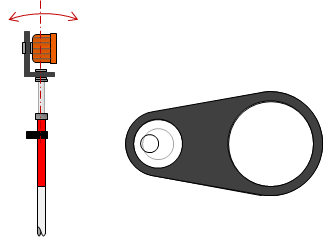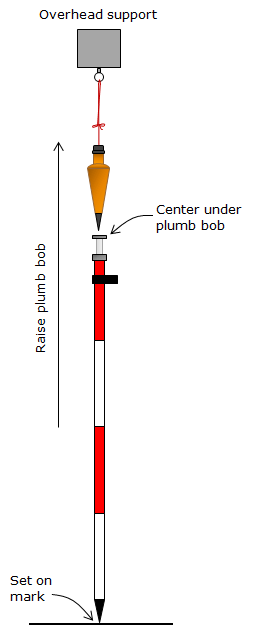1. Sources

We'll identify and examine major errors within each of the three sources.

a. Personal

(1) Centering

This involves how accurately the operator can center the TSI or tribrach vertically over the ground mark. If using a hand held prism pole, how carefully the rodperson holds the bubble centered.

(2) Height measurement

If the TSI will be used for trigonometric leveling or topo data collection then the heights of the instrument and prism must be measured.

(3) Atmospheric conditions determination

Temperature and barometric pressure must be obtained for the time of measurement. If not available, then the operator should record the settings on the TSI so later on they can be compensated.

b. Instrumental

(1) Leveling bubbles

At the TSI, proper leveling techniques should be used to compensate for the plate bubble being out of adjustment. This is discussed more fully in Total Station Instruments Chapter B.

The prism may be mounted in a tribrach in which case the tribrach bubble must be checked for adjustment. If the prism is mounted on a prism pole, the circular bubble can be checked as on the TSI. The following procedure shown in Fgure B-1 should be done with a pole tripod.

 Step (1) Level the rod using the circular bubble.(a) Step 1 Step (2) Rotate the rod 180°. If the bubble stays centered, it's in adjustment. If it runs...(b) Step 2 Step (3) ...bring it back halfway by releveling the rod. Then, if adjusting the bubble...(c) Step 3 Step (4) ...use the adjusting screws on the bottom to center the bubble.(d) Step 4 Figure B-1 Checking and Adjusting Prism Pole Bubble

If a pole tripod is not available, another way to check and adjust the rod bubble can be done using a plumb bob, Figure B-2.(a) Set Ground Point (b) Ceter Pole Step (1) Suspend a plumb bob from an overhead support. Set a mark beneath the plumb bob. Step (2) Raise the plumb bob just high enough to clear the pole. Place the pole on the mark and center under the plumb bob. Step (3) If the bubble isn't centered, use its adjusting screws to correct it. Figure B-2 Alternate Bubble Check and Adjustment

(2) Optical plummet

Optical plummets on the TSI and prism tribrach are used to orient the instrument vertically over its ground mark. These should be checked and adjusted as necessary.

The process for checking optical plumment adjustment are described in Total Station Instruments Chapter B.

(3) Manufacturer's Stated Accuracy (MSA)

Each TSI has an inherent random error in distance measurement. This is the Manufacturer's Stated Accuracy (MSA) and is specified in the instrument manual. It is expressed as a two part uncertainty: (1) a constant, and, (2) a proportion based on distance. An example is an MSA of ±(2mm + 3ppm). Every distance measured with this TSI would have an expected error of:

Constant = ±2 mm x (39.37 in/1 m) x (1 m/1000 mm) x (1 ft/12 in) = ±0.006 ft

The proportional error will increases as do distances.

In a 100.00 ft distance, the expected proportional error is: 100.00 ft x (±3/1,000,000) = ±0.0003 ft

For 1000.00 ft: 100.00 ft x (±3/1,000,000) = ±0.003 ft

(4) Combined constant

The points of signal origin and signal reflection may not be on the vertical axes used to orient the equipment over the ground points. Most surveyors are familiar with a prism offset and how it is affected by the mounting system, Figure B-3.Figure B-3 Mounting Offsets

Additionally, because glass is denser than atmosphere the lightwave is slowed as it travels through the prsim, increasing the measured distance. A manufacturer reports this combined effect as the prism constant or offset.

The TSI is also subject to an offset: the signal is generated internally then optically made to coincide with the line of sight. The slope distance is the sum of the measured distance and the instrument and prism constants, Figure B-4.Figure B-4 Combined Constant

 S = S'+(CI+CP) Equation B-1 S: corrected slope distance S': measured slope distance         CI: Instrument offset CP: Prism offset

(CI+CP) is referred to as the Combined Constant. Using a matched TSI & prism combination and inputting the prism constant corrects the combined effects. However, over time the TSI may lose calibration and rough prism handling require determination of a new constant to use. The most effective way to so this is using an NGS calibration baseline and following NGS' calibration procedure. Baseline data and procedures are available at http://www.ngs.noaa.gov/CBLINES/calibration.html on the NGS web site.

This is a very time and computation intensive process. A simple and accurate method for checking the combined constant can be perfomed by setting up and measuring a three point baseline.

Step (1) Set the prism constant to 0 on the TSI and apply appropriate atmospheric settings before making any measurements.

Step (2) On open relatively flat terrain set three points (A, B, & C) on a straight line. The outer two points (A & C) should be 150 to 300 feet apart; the inner point (B) should be near the midpoint of the line. Point B does not have to be at the exact midpoint but all three points must be on a straight line, Figure B-5.Figure B-5 Calibration Baseline

Step (3) Set up the TSI at point A, Figure B-6, and measure the distance to C multiple times. The prism should be rigidly mounted (not hand held) and at approximately the same elevation as the TSI. For best accuracy, measure distances in the direct and reverse positions. Compute and record the average distance AC.Figure B-6 First TSI Position

Step (4) Set up the TSI at point B, Figure B-7, and measure the distances to points A and C multiple times. Compute and record the average distances BA and BC.Figure B-7 Second TSI Position

Step (5) The combined constant, k, affects all three measurements. Since the TSI was set to a 0 prism constant we expect a disparity between the total distance AC and the sum of its parts BA+BC:

 AC+k = (BA+k) + (BC+k)  AC+k = BA + BC + 2k → k = AC - (BA + BC) Equation B-2

Step (6) The calibration should be run independently a second time to check for consistency.

Step (7) When done, k should be used in place of the prism constant for that particular TSI and prism combination.

If different prisms are used with a single TSI, each combination should have its own combined constant determined, recorded, and applied.

(5) Prism height

For as simple an instrument as is the prism pole, it can lead to some problems. We have already discussed the pole's bubble; what about its height?

Prism pole height does not affect horizontal distance determination. Remember that the TSI uses a zenith angle with the slope distance to compute the horizontal distance. Prism height doesn't matter since raising or lowering it will change both zenith angle and slope distance but still result in the same horizontal distance.

Prism height can come into play in topographic surveys where elevations are determined trigonometrically using instrument and reflector heights. These are discussed in the Trigonometric Leveling chapter in the Elevations topic.

c. Natural

(1) Atmospheric conditions

Electro-optical EM signals are affected by atmospheric pressure and temperature. TSIs are generally standardized at a specific temperature and pressure. When measurement conditions deviate from either then a proportional correction must be applied. This is normally done by the TSI after the operator provides it some necessary information (temperature and pressure or the actual correction factor).

Typical equations for the atmospheric correction are:

 EnglishEquation B-3 PE: Pressure, in Hg TE: Temperature; ºF MetricEquation B-4 PM: Pressure, mm Hg TM: Temperature; ºC

Different manufacturers may use slightly different standardization conditions so refer to the user manual for the correction equations for a partucular instrument.

To apply the correction:

 D = D'x(1+corr'n) Equation B-5 D: Corrected distance D': Uncorrected distance corr'n: correction as ppm

It was mentioned under Personal errors that if the temperature and pressure are unknown at the time of measurement, the TSI settings should be recorded. Later on conditions at measurement time can be determined from the internet or local radio/TV station. The measurements can then be "uncorrected" for the recorded field conditions and "re-corrected" using the appropriate data.

(2) Refraction and curvature

The EM signal path is bent, refracted, as it moves through the atmosphere. The degree of refraction depends on atmospheric density and the signal's direction through it. This affects the zenith angle because it is measured from the vertical to a line tangent to the signal path at the TSI, shown as the dashed line in Figure B-10.

Over long distances earth curvature must be taken into account. Vertical lines at the TSI and reflector are not parallel so the "horizontal" distance is actually a chord distance whose endpoints are at the same elevation as the TSI.This chord is not perpendicular to the vertical at either the TSI or prism.

Figure B-8 shows the combined effect of refraction and curvature.Figure B-8 Curvature and Refraction

The equations for reducing slope to horizontal and vertical distances incorporating refraction and curvature are:Equation B-6Equation B-7 H: Horizontal distance V: Vertical distance S: Slope distance Z: Zenith angle k: refraction constant R: Earth radius

The refraction constant is a percentage of the earth radius. At most elevations a k value of 0.142 is used (14.2% of radius). At higher elevations where the atmosphere is thinner and less refraction is experienced a k value of 0.071 is typically used. Either way, refraction is the smaller effect of the two.

Note that these two equations are modified forms of Equations A-2 and A-3 with a second term (in brackets) added to each for refraction and curvature. The size of the second terms depend on the line length and zenith angle. As distance decreases, so do the second terms until the results of Equations B-6 and B-7 equal those of Equations A-2 and A-3.

Most TSIs provide the option to apply refraction and curvature corrections as measurements are made. Data collection software also provide a correction option as does some desktop surveying software. Care must be exercised so that the correction is not applied multiple times. The safest approach is to turn on the correction in the TSI and not in the data collection or desktop software.

(3) Atmospheric anomalies

The atmospheric correction and refraction are based on an assumption that the atmosphere is either uniform or changing uniformly along the signal path. This is not always the case. Consider the atmosphere immediately above an asphalt surface on a sunny day - the heat emitted by the surface causes a local atmospheric anomaly which affects the signal path. Almost any surface will have a boundary layer of atmospheric disturbance if it has been subject to sunlight.

Their effect can only be minimized or eliminated by not measuring through them. This isn't always practical. For example many Public Land Survey (PLS) corners are located in roads. Not only might the surveyor have to deal with an anomaly at a PLS corner, he may be measuring along the pavement to an adjacent corner half a mile away.

Strategies can include measuring on a cloudy day, late at night, or early morning. Depending on the surface, increasing the TSI and/or prism height may raise the signal sufficiently above the boundary layer.

2. Types

While we didn't identify all the errors affecting electro-optical distance measurement accuracy, we've hit on most of the major ones in each source. Let's categorize those based by their behavior or Type, Table B-1:

 Table B-1 Source Error Type Personal Centering Random Height measurement Systematic Atmospheric conditions determination Systematic Instrumental Bubbles: TSI and rod Systematic Optical plummet Systematic Manufacturer's Stated Accuracy Random Combined constant Systematic Reflector height Systematic Natural Atmospheric conditions Systematic Refraction and curvature Systematic Atmospheric anomalies Random